International
Tables for
Crystallography
Volume F
Crystallography of biological macromolecules
Edited by M. G. Rossmann and E. Arnold

International Tables for Crystallography (2006). Vol. F, ch. 13.4, p. 285   | 1 | 2 |

## Section 13.4.8. Interpolation

M. G. Rossmanna* and E. Arnoldb

aDepartment of Biological Sciences, Purdue University, West Lafayette, IN 47907-1392, USA, and  bBiomolecular Crystallography Laboratory, CABM & Rutgers University, 679 Hoes Lane, Piscataway, NJ 08854-5638, USA
Correspondence e-mail:  mgr@indiana.bio.purdue.edu

### 13.4.8. Interpolation

| top | pdf |

Some thought must go into defining the size of the grid interval. Shannon's sampling theorem shows that the grid interval must never be greater than half the limiting resolution of the data. Thus, for instance, if the limiting resolution is 3 Å, the grid intervals must be smaller than 1.5 Å. Clearly, the finer the grid interval, the more accurate the interpolated density, but the computing time will increase with the inverse cube of the size of the grid step. Similarly, if the grid interval is fine, less care and fewer points can be used for interpolation, thus balancing the effect of the finer grid in terms of computing time. In practice, it has been found that an eight-point interpolation (as described below) can be used, provided the grid interval is less than 1/2.5 of the resolution (Rossmann et al., 1992). Other interpolation schemes have also been used (e.g. Bricogne, 1976; Nordman, 1980; Hogle et al., 1985; Bolin et al., 1993).

A straightforward `linear' interpolation can be discussed with reference to Fig. 13.4.8.1(in mathematical literature, this is called a trilinear approximation or a tensor product of three one-dimensional linear interpolants). Let G be the position at which the density is to be interpolated, and let this point have the fractional grid coordinates Δx, Δy, Δz within the box of surrounding grid points. Let 000 be the point at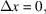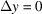,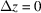. Other grid points will then be at 100, 010, 001 etc., with the point diagonally opposite the origin at 111.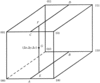Figure 13.4.8.1 | top | pdf |Interpolation box for finding the approximate electron density at G(Δx, Δy, Δz), given the eight densities at the corners of the box. The interpolated value can be built up by first using interpolations to determine the densities at A, B, C and D. A second linear interpolation then determines the density at E (from densities at A and B) and at F (from densities at C and D). The third linear interpolation determines the density at G from the densities at E and F. [Reproduced with permission from Rossmann et al. (1992).]

The density at A (between 000 and 100) can then be approximated as the value of the linear interpolant of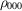and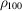: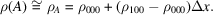Similar expressions for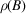,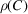and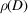can also be written. Then, it is possible to calculate an approximate density at E from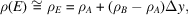with a similar expression for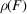. Finally, the interpolated density at G between E and F is given by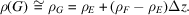Putting all these together, it is easy to show that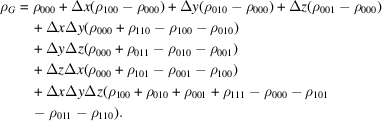### References

Bolin, J. T., Smith, J. L. & Muchmore, S. W. (1993). Considerations in phase refinement and extension: experiments with a rapid and automatic procedure. American Crystallographic Association Annual Meeting, May 23–28, Albuquerque, New Mexico, Vol. 21, p. 51.Google Scholar
Bricogne, G. (1976). Methods and programs for direct-space exploitation of geometric redundancies. Acta Cryst. A32, 832–847.Google Scholar
Hogle, J. M., Chow, M. & Filman, D. J. (1985). Three-dimensional structure of poliovirus at 2.9 Å resolution. Science, 229, 1358–1365.Google Scholar
Nordman, C. E. (1980). Procedures for detection and idealization of non-crystallographic symmetry with application to phase refinement of the satellite tobacco necrosis virus structure. Acta Cryst. A36, 747–754.Google Scholar
Rossmann, M. G., McKenna, R., Tong, L., Xia, D., Dai, J.-B., Wu, H., Choi, H.-K. & Lynch, R. E. (1992). Molecular replacement real-space averaging. J. Appl. Cryst. 25, 166–180.Google Scholar